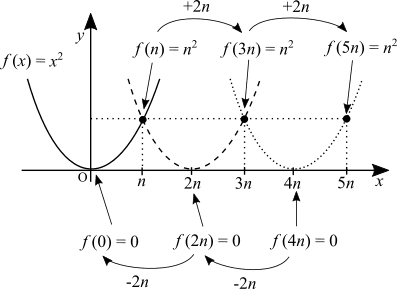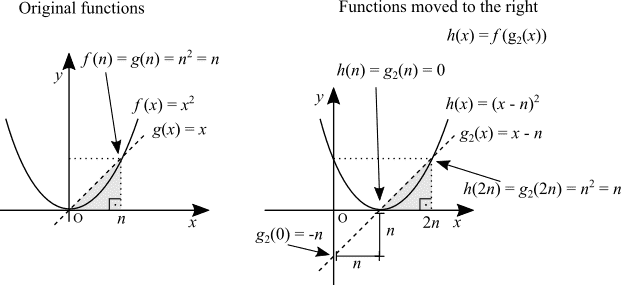# Moving the graph to the right -- What do you think?

0kelvin
I'm studying calculus alone with textbooks. The part about moving the graphs to the right or to the left struck me because they just have a list of rules, properties and make you relate the graph with the corresponding equation. I know what is the rate of change and I thought I could do better than the textbook.

I vectorized this to explain why: f(x - n) moves the parabola to the right.Not satisfied I though. f(x - 2) does remind me of the concept of a composite function. Can I draw something to explain this and relate it to the rate of change?•mcastillo356

Gold Member
With a graph described with an equation ##f(x,y)=0## given, another graph
$$f(x-a,y-b)=0$$
is a translation of that graph with vector (a,b) on x-y plane. For an example say (0,0) is on the original graph, it is translated to (a,b) on the new one.

Last edited:
Homework Helper
Gold Member
2022 Award
I'm studying calculus alone with textbooks. The part about moving the graphs to the right or to the left struck me because they just have a list of rules, properties and make you relate the graph with the corresponding equation. I know what is the rate of change and I thought I could do better than the textbook.
Take a function that is zero everywhere except the origin:$$f(x)=\begin{cases} 1 & x = 0 \\ 0 & x \ne 0 \end{cases}$$Now define ##g(x) = f(x -2)##. Note that ##g(2) = f(0) = 1##, hence:$$g(x)=\begin{cases} 1 & x = 2 \\ 0 & x \ne 2 \end{cases}$$And we see that ##g(x)## is ##f(x)## moved to the right.

•mcastillo356
Homework Helper
Gold Member
Here's a related example from physics: a traveling wave disturbance on a string.

Suppose a disturbance has a profile F(x) along a string.
[In physicist's notation...]
F(x-vt) describes that disturbance translating (traveling without distortion) to the right with constant velocity v.

At t=0, consider the disturbance at the string location x=1: F(1).
After a time t, F(1)=F(x-vt) where 1=x-vt.
Since t increases, x must increase to keep x-vt=1. (Indeed, x=vt+1.)
...and similarly for other locations.
Thus, the disturbance moves to the right.

See https://www.desmos.com/calculator/bjt6dleg5h
from
https://www.physicsforums.com/threa...mean-in-the-wave-equation.836348/post-5254546